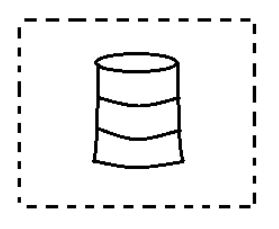QuestionAnswers

# In the figure, you see the frame of a lampshade. It is to be covered with a decorative cloth. The frame has a base diameter of 20cm and a height 30cm and a margin of 2.5cm is to be given for folding it over the top and bottom of the frame. How much cloth is required for making lampshades?Hint:: As per figure, the shape of the frame of the lampshade is cylindrical. In this question, we have to calculate the total cloth required to cover the lampshade. So, to calculate this we have to use the formula of the curved surface area of a cylinder. For getting a curved surface area of cloth, we will have to multiply the circumference of the lampshade with the height of the cloth required. So, we need to calculate the total height of the cloth required to make the frame of the lampshade (cylinder) that will be obtained by adding the upper margin & lower margin of cloth (needed to be folded from both upper & lower side) to the height of the cylinder (frame of lampshade) to get the curved surface area of cloth required.

Complete step by step solution:
The diameter of the frame of the lampshade(cylinder) is $20cm$.
The height of the frame of the lampshade is $30cm$.
Cloth taken extra for upper & lower margins for frame - $2.5cm$
So, the radius will be $R = \dfrac{{Diameter}}{2} = \dfrac{{20}}{2} = 10cm$
As the diameter is twice the length of the radius so to calculate the radius, we have to divide the above diameter by two.
So, total height for lampshade = height of frame + height of cloth for folding from upper margin & lower margin
$= 30 + 2.5 + 2.5$
This value will be equals to,
$= 35cm$
To calculate the total height, we have to add the height given to the height of the margin (upper and lower margin both).
So, we have to calculate the curved surface area of cloth that will be obtained by its formula:
Curved Surface Area $= 2\pi rh$
Hence, Area of the cloth required for lampshade (cylinder)
Curved Surface Area $= 2\pi rh$
As we know the value of $\pi$ is $\dfrac{{22}}{7}$, On substituting it,
$= 2 \times \dfrac{{22}}{7} \times 10 \times 35$
$= 44 \times 50$
On simple multiplication,
$= 2200c{m^2}$

$\therefore$ The total area of cloth required to decorate the lampshade will be $= 2200c{m^2}$.

Note:
Read the question very attentively first because, in kind of real-life problems, there is a high chance of getting confused whether a curved surface area or total surface area should be considered to get the area of cloth required. Do the calculations carefully to avoid silly mistakes even after knowing the whole procedure to do the sum. This method is very easy among all other methods that are used to calculate the problem of the type because here you just need to calculate the height of cloth required according to the question & then apply the formula of the curved surface area of the cylinder to get the answer. Lastly, don’t ever forget to give an answer along with its unit (here $c{m^2}$) because of a no. without a unit is meaningless.